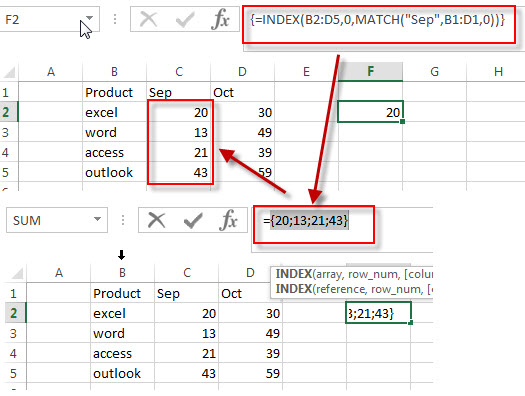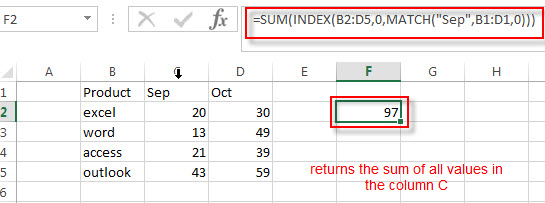# How to SUM a Column with Lookup value in a Range

We have described that how to lookup value and retrieve the entire column in the previous post. And this post will guide you how to get the sum of the entire column with lookup value in a range in excel.

## SUM Column with Lookup Value

If you want to lookup value in a range and then retrieve the entire row, you can use the MATCH function within the INDEX function.  For example, if you want to search “Sep” string in the range B1:D1, then return the entire column of a matched value in the range B2:D5, you can write down the following array formula:

`=INDEX(B2:D5,0,MATCH("Sep",B1:D1,0))`If you want to return the sum of the entire column, you need to use the SUM function in combination with the INDEX function and MATCH function. To get the sum of all values in a column that returned by the above INDEX/MATCH formula, you can use the following formula:

`=SUM(INDEX(B2:D5,0,MATCH("Sep",B1:D1,0)))`The INDEX/MATCH formula returns an array result that contain all values in the matching column like this:

`{20;13;21;43}`

The SUM function returns the sum of all elements in the array returned by the INDEX/MATCH functions. It returns 97.

### Related Formulas

• If you want to lookup entire row and then return all values of the matched row, you can use a combination of the INDEX function and the MATCH function to create a new excel array formula.
• Extract the Entire Column of a Matched Value
If you want to lookup value in a range and then retrieve the entire row, you can use a combination of the INDEX function and the MATCH function to create a new excel formula..…
• Lookup the Value with Multiple Criteria
If you want to lookup the value with multiple criteria in a range, you can use a combination with the INDEX function and MATCH function to create an array formula.…
• Lookup the Next Largest Value
If you want to get the next largest value in another column, you can use a combination of the INDEX function and the MATCH function to create an excel formula..
• Find the nth Smallest Value
You can use the SMALL function to get the 1st, 2nd, 3rd, or nth smallest value in an array or range. Also you can use the SMALL function within the INDEX function to extract the relative value of the same row…

### Related Functions

• Excel SUM function
The Excel SUM function will adds all numbers in a range of cells and returns the sum of these values. You can add individual values, cell references or ranges in excel.The syntax of the SUM function is as below:= SUM(number1,[number2],…)…
• Excel INDEX function
The Excel INDEX function returns a value from a table based on the index (row number and column number)The INDEX function is a build-in function in Microsoft Excel and it is categorized as a Lookup and Reference Function.The syntax of the INDEX function is as below:= INDEX (array, row_num,[column_num])…
• Excel MATCH function
The Excel MATCH function search a value in an array and returns the position of that item.The syntax of the MATCH function is as below:= MATCH  (lookup_value, lookup_array, [match_type])….